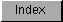```
The speed of the blitter depends entirely on which  DMA channels  are
enabled.  You might be using a  DMA channel  as a constant, but unless it
is enabled, it does not count against you.  The minimum blitter cycle is
four ticks; the maximum is eight ticks.  Use of the A register is always
free. Use of the B register always adds two ticks to the blitter cycle.
Use of either C or D is free, but use of both adds another two ticks.
Thus, a copy cycle, using A and D, takes four clock ticks per cycle; a
copy cycle using B and D takes six ticks per cycle, and a generalized bit
copy using B, C, and D takes eight ticks per cycle. When in  line mode ,
each pixel takes eight ticks.

The system clock speed for NTSC Amigas is 7.16 megahertz (PAL Amigas 7.09
megahertz). The clock for the blitter is the system clock.  To calculate
the total time for the blit in microseconds, excluding setup and DMA
contention, you use the equation (for NTSC):

n * H * W
t = ---------
7.16

For PAL:

n * H * W
t = ---------
7.09

where t is the time in microseconds, n is the number of clocks per cycle,
and H and W are the height and width (in words) of the blit, respectively.

For instance, to copy one bitplane of a 320 by 200 screen to another
bitplane, we might choose to use the A and D channels.  This would require
four ticks per blitter cycle, for a total of

4 * 200 * 20
------------ = 2235 microseconds.
7.16

These timings do not take into account blitter setup time, which is the
time required to calculate and load the blitter registers and start the
blit.  They also ignore DMA contention.

```